# Greedy Algorithms in C++ (10 Popular Problems with Solutions)

With each passing day, programmers are trying to devise solutions to tackle complex problems by including daily life instances. Even in interviews, major coding questions have a connection with our day-to-day lives. Many algorithms are being created to find solutions as quickly as possible. One such algorithm is the Greedy Algorithm. It is mostly used when you are looking for an immediate solution and do not care about future consequences.

Algorithms like Divide and Conquer, and Greedy are gaining popularity in interviews. The approach toward solving a question using the Greedy Algorithm revolves around the concept of finding the locally optimized solution in the minimum time frame. Questions revolving around this concept are majorly being asked, so you need to understand all about these. In this blog, we'll know everything about these algorithms, their structure, advantages, disadvantages, and the top 10 problems with their C++ code. So, let's dive into this and understand all about them.

## What is a Greedy Algorithm?

The optimization problems of maximizing/minimizing a particular quantity are solved by a special algorithm called Greedy Algorithm. Now, why do we solve only these types of questions with this? Well, it is not a hard & fast rule to solve only these sorts of questions, but the important thing is, that it helps in making immediate choices. When we use the Greedy Algorithm, we are looking only for a locally optimized solution and not a global one, which mostly puts us in the cage. In some cases, this approach may give the right solution initially, but towards the end, it may look unnecessary.

This approach has its merits and demerits. Although, it is a good way of solving optimization problems, still in some cases it overlooks some conditions. Therefore, it is important to observe each step while working with this algorithm to get the most optimal solution that follows the provided dataset.

Let's understand this algorithm with an example.

### Example of Counting Coins using Greedy Algorithm

We are given certain coins valued at \$1, \$2, \$5, and \$10. Now you need to count the specified value using these coins.

Case 1: \$16

The greedy procedure will be like this.

• Firstly, we'll take 1 coin for \$10. So, now we are less by \$6.
• Now, we'll take 1 coin of \$5 and later, 1 coin of \$1.

Why did we choose the \$10 coin first, we could have chosen other coins as well. Because we're Greedy. We take the coin that has greater value. In this case, the answer came out to be just fine. What if we take another case?

Case 2: \$17

The procedure will be as follows:

• Take 1 \$10 coin. Now, we are less by \$7.
• We do not have any other choice, than choosing 7 coins of \$1 each.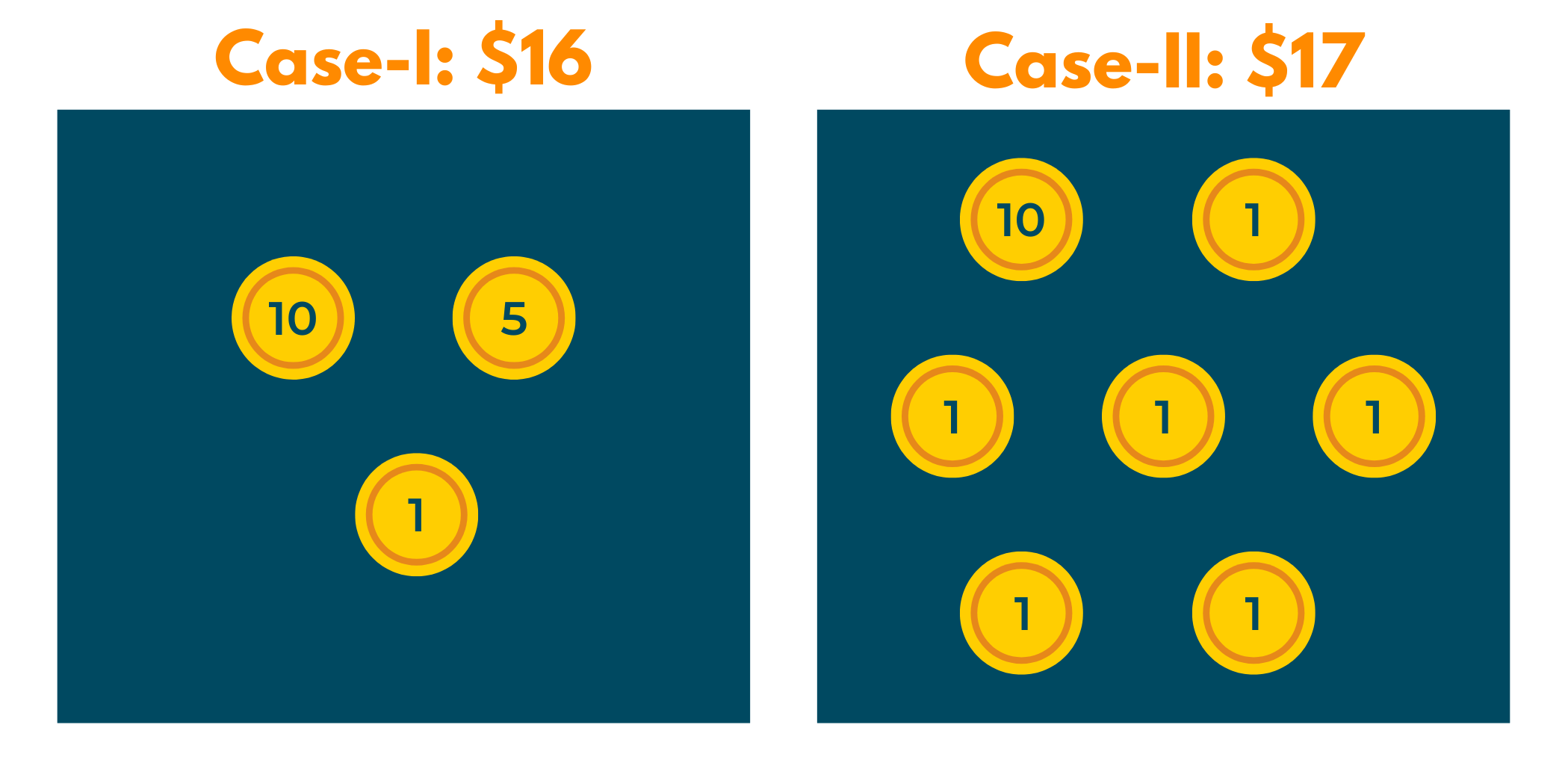Now, this is not a very optimal idea. That's where the greedy algorithm fails. Hence, we can say that using these is great for immediate solutions but not for a globally optimized solution.

### Basic Structure of a Greedy Algorithm

Below we've mentioned a few attributes of a Greedy Algorithm that helps you know whether the approach you follow is Greedy or not:

1. We are given an ordered list of resources like coins, tasks, etc.
3. Later, we keep on adding the greater valued resources to reach the optimized solution.

1. This is easier to implement & understand.
2. The time complexity of this is significantly less.
3. These can be easily used for finding an optimized solution to a complex problem.

The only demerit of following this approach is that we overlook the importance of a globally optimized solution. In most cases, we generally get a locally optimized solution and that puts us in a problem while looking at the whole dataset.

### Components of Greedy Algorithm

The general components of this approach have been mentioned below:

1. Candidate Set: solution to the problem that has been created from the dataset.
2. Selection Function: a function that decides whether the element can be added as a solution or not.
3. Feasibility Function: checks whether the elements selected in the selection function & candidate set are feasible or not.
4. Objective Function: assigns value to the solution.
5. Solution Function: used to intimate the reaching of complete function.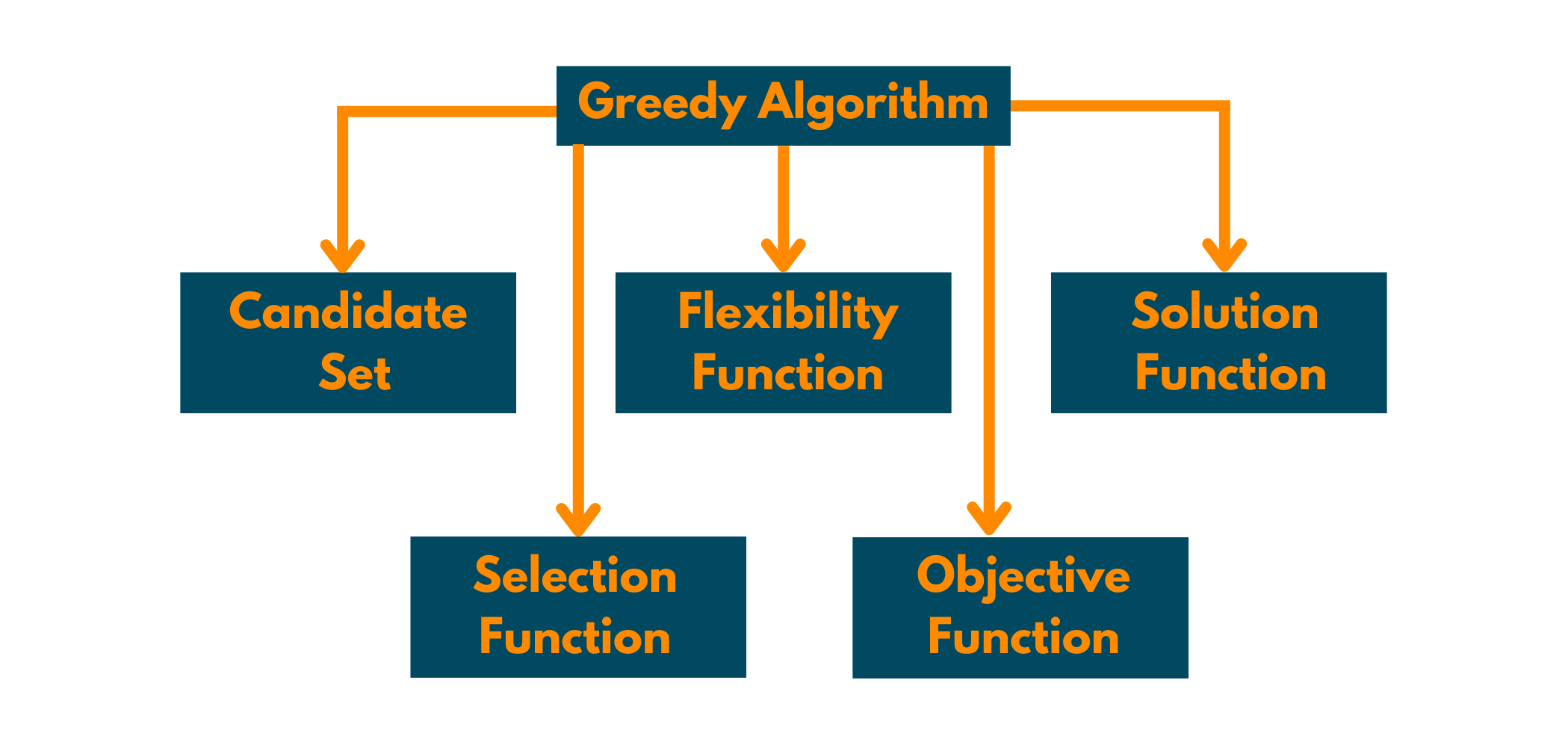### Applications of Greedy Algorithm

1. Finding the shortest path using Dijkstra.
2. Finding a minimum spanning tree using Prim's algorithm or Kruskal's algorithm.
3. Solving fractional knapsack problem.

## Top 10 Greedy Algorithm Problems with C++ Code

Since you've understood the basics of Greedy Algorithms, let's undergo the Top 10 problems that are frequently asked in technical rounds. For your ease, we've given the approach as well as the C++ code of the problems.

### 1) Activity Selection Problem

Problem Statement:  You are given n activities with their beginning and ending times. Assuming that a person can work only on 1 activity at a time, find the maximum number of activities he can perform in a minimum time.

Approach towards Solution: As we're solving it using the Greedy approach, it is quite obvious to choose an activity that gets completed in a lesser time. Why? This is because then we'll be able to do many tasks in a specified time rather than focusing on doing just one heavy-timed task. Therefore, we must choose the tasks in non-decreasing order.

1. Begin the solution by sorting the given arrays in ascending order using the sorting technique of your choice.
2. Keep in mind that to begin doing the activities, the person has to start with the first one. It means that no matter what inputs we've been given, the first activity is always implemented. Why? Because if you're going to do a single activity, it does not matter which one you do!
3. Understand that the person will be able to take up new activity only after completing the previous one. If the person is already doing the previous activity at that time, reject the next one and move on. So, select the next one if its start time is greater or equal to the finish time of the previous activity.

Example:

Input: start[]={10,12,20} ; finish[]={20,25,30}

Output: {10,20}, {20,30}

Explanation: We've already understood that the first task gets implemented whatsoever. So, the {0} charge gets added to the solution array. Moving on, the finish time of 0th work is compared to the start time of {1}. Now, since it is less than the finish time of the previous one, we move on. Again the comparison happens and the {2} task gets selected.

Implementation with C++ Code:

```//C++ Program For Activity Selection Problem
#include
#include <bits/stdc++.h>
using namespace std;

//Function to sort the array according to smallest value of second element in array
static bool Compare(vector<int>& a, vector<int>& b){
return a<b;
}

//Function to print total activities
void printActivties(vector<pair<int,int>>& arr){
cout<<"Activities that a single Person Can Select Are : "<<endl;
for(int i=0;i<arr.size();i++){
cout<<arr[i].first<<","<<arr[i].second<<endl;
}
cout<<endl;
}
//Function to select activities
int activitySelection(vector<int> start, vector<int> end, int n,vector<pair<int,int>>& activties)
{
//Storing start and end array in a 2d array
vector<vector<int>> arr;
for(int i=0;i<n;i++){
arr.push_back({start[i],end[i]});
}

//Sorting Array according to ending time
sort(arr.begin(),arr.end(),Compare);

//There will be atleast one activity
int Total_Activity = 1;

//Activity that is ending first
int endValue = arr;

//Pushing the first activity
activties.push_back({arr,arr});

for(int i=1;i<n;i++){
//if start time of ith activity is greater than ending time of previous taken activity then update ending time, increase the value of activity and store the answer in activities array
if(arr[i]>endValue){
endValue = arr[i];
Total_Activity++;
activties.push_back({arr[i],arr[i]});
}
}

}

int main() {
//Adding elements in array of Starting and Ending duration for activity
vector<int> start = {10,15,30,45,50,65};
vector<int> end =   {20,25,40,60,55,80};

//Size of the array
int n = start.size();     //Total activites array that a single person can select
vector<pair<int,int>> activities;

//Funtion Call for finding total activities to select by single person
int totalActivity = activitySelection(start,end,n,activities);

//Printing the activities that can select
cout<<"Total Activities Are : "<< totalActivity <<endl;
printActivties(activities);

return 0;
}
```

Output:

```Total Activities Are : 4
Activities that a single Person Can Select Are :
10,20
30,40
50,55
65,80
```

### 2) Best Time to Buy and Sell Stock

Problem Statement:  You are given array prices [] which contain the price of a stock on some days. You have to choose one day for buying the stock and a different one for selling it. Return the days on which you buy and sell the stock.

Approach towards Solution: In this question, you need not know anything about stocks, the approach we're providing is easy enough to understand even for beginners. Now, it's quite obvious that when you want to purchase anything, you want to pay the least price you can. Similarly, while selling the same stuff, you'll want to increase your profits by selling it at a larger price. Thus, you're greedy to increase your profits. So, let's see how you'll go with this question:

1. Here, sorting won't work as you have to give the number of the day. So, you will first find the minimum value of the stock from prices[]
2. The index of the minimum value will become the day you buy your stock.
3. To sell the stock, you have to check the value of the stock days after you've purchased the stock.
4. Hence, run a loop till the end starting from buyingDay, and find the maximum value of the stock in the remaining array.
5. Now, subtract the value of buyingDay from sellingDay, and voila! You've got the answer!

Example:

Input: {7,1,5,3,6,4}

Output: 5

Explanation: As we've mentioned in the approach, first you'll find the least element, which is 1, here. Now, the buyingDay becomes 1. After that, we search for the maximum value of stock in the days following 1. So, the sellingDay becomes day having 6 value. Now, we find the difference between both and get 5 as our output. Take a look at the below illustration.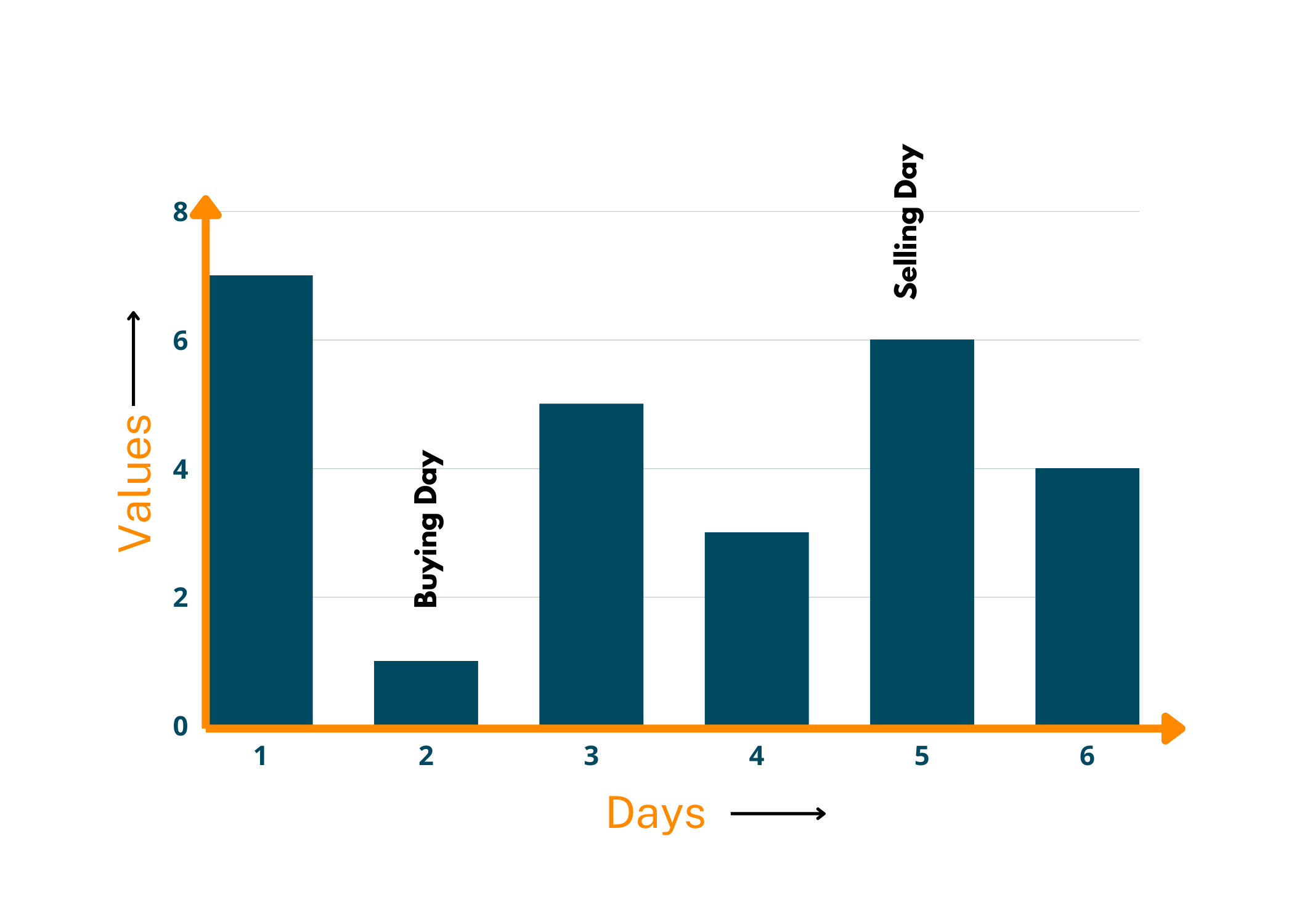Implementation with C++ Code

```//C++ Program For Best Time to Buy and Sell Stock
#include
#include<bits/stdc++.h>

using namespace std;

int main() {
//Implementing the array for prices on ith days of stock
int n = 6;
vector<int> prices = {7,1,5,3,6,4};

//for storing best days to buy and sell stock
pair<int,int> ans;
//For storing maximum profit
int maxProfit = 0;
//For storing minimum purchase of stock
int ind;

for(int i=0;i<prices.size();i++){

//if we get min price for buying than earlier then we update minBuy and its index
ind = i;
}

//Finding maximum profit by checking selling at ith day prices with minBuy
ans = {ind,i};      // storing the days of buying and selling
}
}

//Printing the best time to buy and sell stock and its maximum profit
cout<<"Best Time to buy on day "<<ans.first+1<<" and sell on day "<<ans.second+1<<endl;
cout<<"Maximum Profit Will be "<<maxProfit;
return 0;
}
```

Output:

```Best Time to buy on day 2 and sell on day 5.
Maximum Profit Will be 5
```

### 3) Job Sequence Problem

Problem Statement: You are given an array where you've been given certain jobs, their deadline, and the profit you'll earn upon completing them on time. Every job takes at least one unit of time. You have to return the maximum profit you can earn by completing jobs in a certain time.

Approach towards Solution: Since we need to complete maximum jobs in minimum time, we'll go with the greedy approach. We'll sort the jobs in decreasing order of their profits. Now, we'll traverse each job and check its deadline. If in our array, there is a space empty to complete that particular job before time or even in that time, we'll take it up and add its profit to our result variable. Otherwise, we'll move on to the next one. Follow the below steps:

1. Sort the jobs in ascending order of their profits.
2. Start traversing the jobs one by one.
3. If at a job, you've got a time slot empty and its profit is more, you'll do that job and add its profit to your result variable.
4. If not, you'll move over to the next one.

Example:

Input: {{4,20}, {1,10},{1,40}, {1,30}}

Output: {c,a}

Explanation: First we sorted the array of elements according to their profits. Now, the first one has the highest profit which is 40 therefore, we took this job and since its deadline is mentioned as 1 we could either do it in 0-time frame or 1. So, we did it in 0. Now, we move forward and see another whose time frame is 1. But, we had to overlook it since we're already doing the job in that (0) timeframe. Now, when we came over to the next one, we see that it could be completed in 4 therefore, either 0,1,2 or 3. So, we took it and overlooked the last one which had only 1 as its timeframe.

Implementation with C++ Code:

```//C++ Program To Find The Maximum Profit in Jobs array with given deadlines
#include<bits/stdc++.h>
using namespace std;

//Sorting Jobs according to descending order of profit
static bool Compare(vector<int>& a,vector<int>& b){
//Larger profit comes before the smaller in array
return a > b;
}

int main() {
//Implementing an array of jobs with deadlines and its profit
int n = 5;
vector<vector<int>> jobs = {{2,100},{1,19},{2,27},{1,25},{1,15}};

//Sorting Jobs according to profit because we want maximum profit so we sort in descending order for           larger profit comes first before smaller one.
sort(jobs.begin(),jobs.end(),Compare);
int done=0;     //For storing total number of jobs done
int totalProfit = 0;       // For storing maximum Profit
vector<bool> complete(n,0);    //For storing completed jobs on ith days

//Iterating through jobs array
for(int i=0;i<n;i++){
int profit = jobs[i];    // Profit of the ith job
//Finding empty days to complete job before the deadline as it 0-based indexing we decrement                    deadline by 1 while iterating
if(complete[j]==false){
done++;      //Incrementing the no.of jobs done
totalProfit += profit;    //Adding profit in total profit
complete[j] = true;     //Marking this day occupied
break;
}
}
}

//Printing answer of total no.of jobs done and its maximum profit
cout<<"Total number of jobs done are : "<< done<<endl;
cout<<"The maximum profit are : "<<totalProfit;

return 0;
}
```

Output:

```Total number of jobs done are : 2
The maximum profit are : 127
```

### 4) Fractional Knapsack Problem

Problem Statement: You are given the weights and values of items. You need to put these items in a knapsack of capacity W in such a way that the final value of the knapsack is maximum. You can break down the items into fractions to attain the most optimal answer.

Approach towards Solution: Since you are asked to find out the maximum value of the knapsack, it should automatically strike to use the Greedy approach. Now, we need to maximize the value of the knapsack within its fixed capacity. It is quite simple that we'll choose that element whose weight is less but whose value is more. Why? Because we need maximum value within the confined weight, if we choose a greater value with lesser weight, we'll automatically be able to put more elements in the knapsack. Thus, attaining the greatest possible value.

Let's see the steps you'll need to follow:

1. You need elements with less weight and more value. So, we'll calculate the ratios of these two for each element. (Ratio = value/weight)
2. Since we need maximum valued elements first, we'll sort these ratios in ascending order.
3. Now, you'll iterate through the elements and check whether the weight of that element is less than or equal to the maximum weight of the knapsack. If yes, add that to your answer. Otherwise, move on.

Example:

Input: {{60,10}, {100,20}, {120,30}}; = 50

Output: 240

Explanation: Now, the knapsack weight is 50 kgs so you need to add elements with weight equal to this. So, we could have taken the 2nd and 3rd elements but that would exclude the 1st one. Hence, we took 1st and 2nd and then took a fractional (2/3rd) part of the 3rd element. Therefore, getting the maximum possible answer. You can also take a look at the below illustration.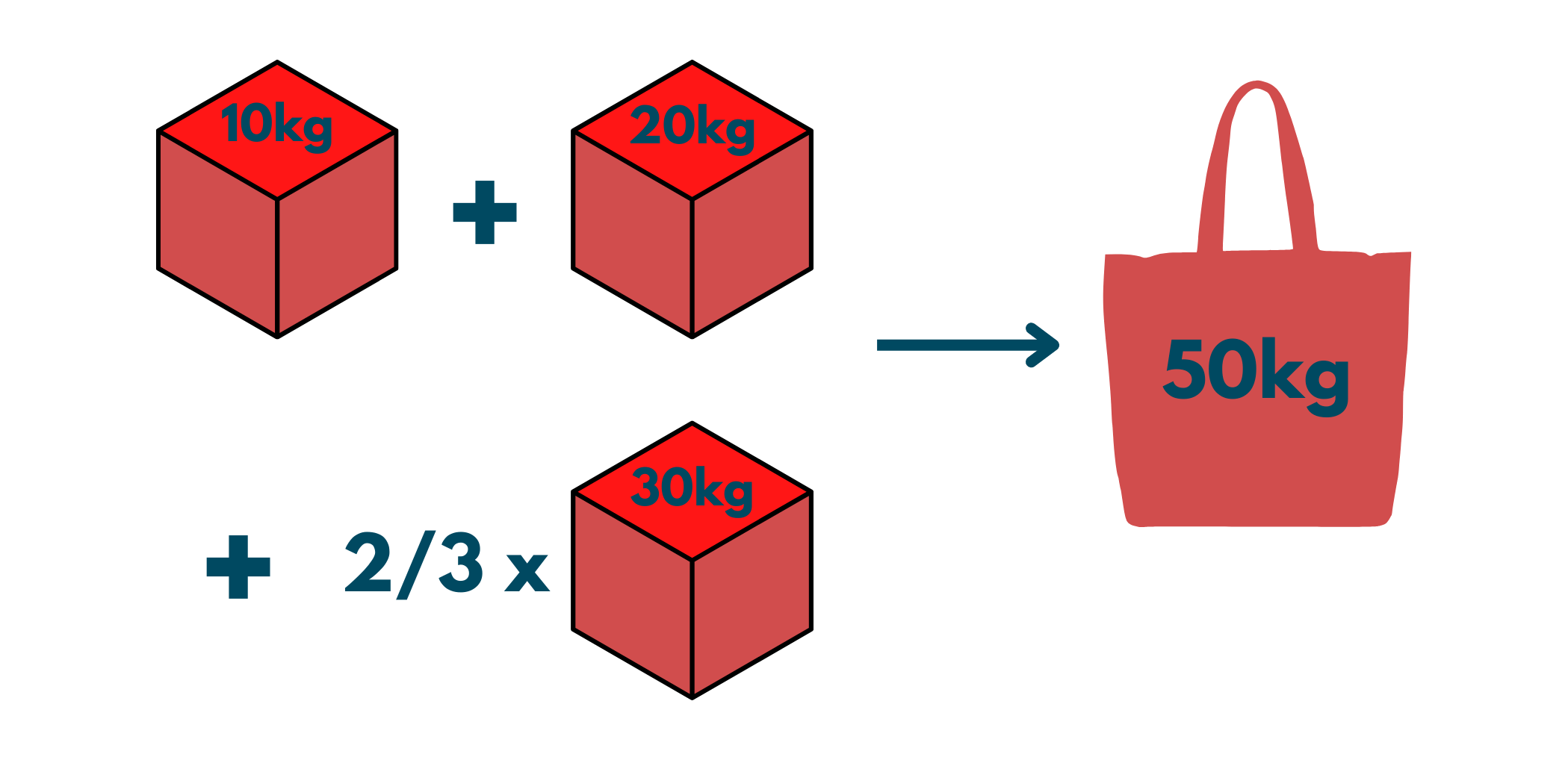Implementation with C++ Code:

```//C++ Program for Fractional Knapsack Peoblem
#include <bits/stdc++.h>
using namespace std;

//Comparison Function to sort array according to value/weight ratio
static bool comp(vector<int>& a,vector<int>& b){
return (double)((double)a/(double)a) > (double)((double)b/(double)b);
}

int main() {
int n = 7;
//Total weights or total capacity
int W = 15;
//Implementing nx2 array containing values and its weights
vector<vector<int>> arr = {{10,2},{5,3},{15,5},{7,7},{6,1},{18,4},{3,1}};

//Sorting array according to value/weight ratio for storing maximum values
sort(arr.begin(),arr.end(),comp);

double totalValues = 0;  // For storing maximum value from the given capacity of sack

//Iterating through given array till the given capacity is not filled
for(int i=0;i<n;i++){
//if we have more capacity in sack than ith weight
if(W>=arr[i]){
totalValues += arr[i];
W -= arr[i];      //decrement capacity by weight we add
}
else{
double fraction = ( (double)arr[i])/((double)arr[i]);
totalValues += fraction*((double)W);
break;
}
}

cout<<"Total Maximum Value of item from the given weight is "<<totalValues;
}
```

Output:

```Total Maximum Value of item from the given weight is 55.3333
```

### 5) Minimum Number of Coins

Problem Statement: We are given a value of and we need to give the change of it in Indian coins. Now the denominations are 1, 2, 5, 10, 20, 50, 100, 200, 500, 2000. The result should contain the least possible change of the given value.

Approach towards Solution: Again, since we need to find the minimum possible number of coins in exchange for the value V, we'll be using Greedy. So, we'll start off with the maximum valued coin which is less than or equal to V. Now, we'll subtract the value of the chosen coin from the given value. For the remaining value, we'll again search for the maximum valued coin and add it to the answer. Follow the steps below:

1. Find the largest valued coin among the given.
2. Add it to the result and subtract from V
3. Repeat the above steps till you find the complete value.

Example:

Input: V=70

Output: 2

Explanation: To get the change of 70, we'll require one note of 50 and another of 20.Implementation with C++ Code:

```#include<bits/stdc++.h>
using namespace std;

int main() {
//Finding minimum number of coins needed to make N
int N = 438;
//Initializing coins array
vector<int> coins = { 1, 2, 5, 10, 20, 50, 100, 200, 500, 2000 };
//Inititalzing ans array to store the number coins needed
vector<int> ans;
int n = coins.size(); // Size of the array
//Looping the coins from the end
for(int i=n-1;i>=0;i--){
//if N is greater than ith coin than loop while N is not less than ith coin
while(N>=coins[i]){
N -= coins[i]; // Decrementing the N while it is not less than ith coin
ans.push_back(coins[i]); //storing the coin
}
}

cout<<"Minimum number of coin needed are "<< ans;
return 0;
}
```

Output:

```Minimum number of coin needed are :-
200 200 20 10 5 2 1
```

### 6) Minimum Platform Problem

Problem Statement: You are given the arrival and departure timings of certain trains. You need to find the minimum number of platforms on the station so that no train clashes with each other's schedules.

Approach toward Solution: In this question also, we need to find the minimum number of platforms, hence we'll use the Greedy approach to find the answer. We'll check the timings of the train and keep a variable result initialized as 0. If anywhere a clash happens, we'll increment the result by 1. So, in the end, we'll get the minimum number of platforms required.

Example:

Input: arr[]={10:20, 12:00}; dep[]={10:50, 12:30}

Output: 1

Explanation: We'll start off with the departure time of the first train, and check whether the next train arrives in between the schedule of the first one. Since here, nothing like that is happening, we'll only have 1 platform.

Implementation with C++ Code:

```//C++ Program For Minimum Platforms Needed at a Station
#include <bits/stdc++.h>
using namespace std;

int minmumPlatforms(vector<int>& arrival, vector<int> departure, int n)
{
//Sorting arrival and departure train in ascending order
sort(arrival.begin(),arrival.end());
sort(departure.begin(),departure.end());
//Atleast one platform is needed
int platforms_needed = 1;
//Storing the minimum platform
int platforms = 1;
//index of the arrival train array starting from 1 index as 0th train is arrived and take platform 1
int i=1;
//Index of the departure train array
int j=0;
//Looping through both the array
while(i<n && j<n){
//if ith train is arrived and jth train is not departed than increment platform and ith index as               the ith train is arrived and take the incremented platform
if(arrival[i]<=departure[j]){
platforms_needed++;
i++;
}
//else If ith train is not arrived and jth train is departed then the space taken by jth train is             empty so we decrement the platform and increment jth index by 1
else if(arrival[i]>departure[j]){
platforms_needed--;
j++;
}

//if platforms needed is increased by total platforms then we update the total platform into                     platforms needed
if(platforms_needed>platforms) platforms = platforms_needed;
}
//Return the min platform needed for a station
return platforms;
}

int main() {
//Total trains
int trains = 6;
//Initializing arrival and departure of the ith trains
vector<int> arrival = {900, 940, 950, 1100, 1500, 1800};
vector<int> departure = {910, 1200, 1120, 1130, 1900, 2000};

//function Call
int minimum_platforms_needed = minmumPlatforms(arrival,departure,trains);

cout<<"Minimum platforms needed for a train at a station is "<<minimum_platforms_needed;
}
```

Output:

```Minimum platforms needed for a train at a station is 3
```

### 7) Gas Station Problem

Problem Statement: You are given 2 integer arrays of gas[] and cost[]. There are gas stations on a circular route. Every station has a particular amount of gas that gets refilled into the vehicle and it also requires a certain fuel amount to reach the next one. You need to find the starting point so you can travel all the stations around the circuit in a clockwise direction.

Approach toward Solution: You need to start off from that gas station that has gas that can take you to the next one and later, around all the stations after visiting each. So, we'll start checking from the first index to check its possibility and move on to the next one.

Example:

Input: gas[]= {1,2,3,4,5}; cost[]= {3,4,5,1,2}

Output: 3

Explanation: You will start off with 3rd indexed gas station. Now, you'll move with 4 liters of gas and reach the next one. So, to reach that you'll spend 1 liter and gain 5. Similarly, you can check for all other gas stations by doing a DRY run.

Implementation with C++ Code:

```// C++ program for Gas Station Problem
#include <bits/stdc++.h>
using namespace std;

int gasStationIndex(vector<int>& gas, vector<int>& cost) {

int deficit = 0; //To store total negative balance before the start index
int balance = 0; // To store the balance of gas left
int start = 0;

for(int i=0;i<gas.size();i++){
//storing balance
balance += gas[i] - cost[i];

//checking balance
if(balance<0){
//adding in deficit if balance is in negative
deficit += balance;
//Update the answer in i+1th index
start = i+1;
balance=0;
}
}

//if addition of deficit and balance is greater or equal than zero then we can complete the circuit            else we cannot complete the circuit so return -1
if(deficit + balance >= 0){
return start;
}
else{
return -1;
}
}

int main() {
//Intializing the amount of gas at ith station in the array
vector<int> gas = {1,2,3,4,5};
//Intializing the cost of gas from ith station to (i+1)th station
vector<int> cost = {3,4,5,1,2};

int ans = gasStationIndex(gas,cost);

//Printing the starting index from which we can complete the circuit
if(ans!=-1){
cout<<"Starting gas station's index that can travel around the circuit : "<<ans;
}
else{
cout<<"Can't travel around the circuit once no matter where you start.";
}
}
```

Output:

```Starting gas station's index that can travel around the circuit : 3
```

### 8) Connect 'n' Ropes with Minimal Cost

Problem Statement: You are given ropes of different lengths. Now, you need to connect those ropes into a single one. Find the minimum cost of joining all the ropes into a single one. Assume that the cost of joining ropes is equal to the sum of their lengths.

Approach toward Solution: The intuition behind this question is that we will add those two ropes first whose cost of joining comes out to be a minimum. Thereafter, we'll again join those two whose cost upon joining is the least. Similarly, we'll iterate through all the ropes. For this, we'll be using a min-heap through a priority queue. Follow the steps below:

1. Use a min-heap in the priority queue.
2. Take the top 2 elements(they're minimum) and add them. The sum will be added to the result.
3. Now, you are left with one less element. Again, follow the same approach.
4. Keep doing these until a single answer is gained.

Example:

Input: {5,4,2,8}

Output: 36

Explanation: Firstly, initialize a variable called Result=0. Remember to add the cost of joining ropes into this variable. Now, we'll connect ropes of lengths 4 and 2. Thus, Result=6. Now, we're left with {5,6,8}.  So, again we'll take the 2 minimum elements from the array and add them. Result=6+11 Now, we'll join the remaining elements that are 11 & 8. So, finally the Result=6+11+19. Therefore, the final result is 36

For a simpler understanding, observe the below illustration.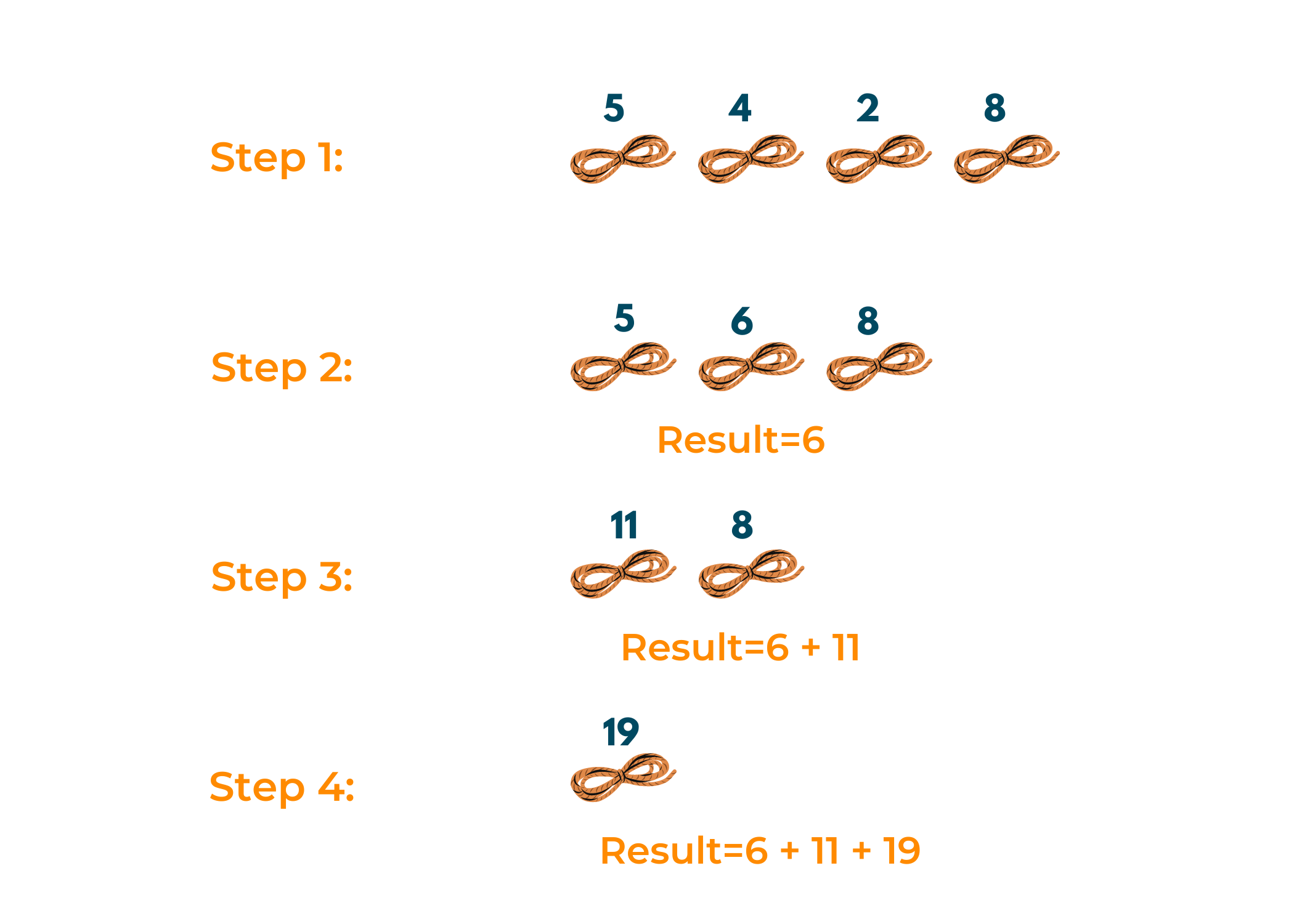Implementation with C++ Code:

```//C++ program for finding minimum cost to combine n ropes
#include
#include<bits/stdc++.h>
using namespace std;

int solve(vector<int> nums, int n) {
//Implementing min heap for finding two smallest ropes
priority_queue<int,vector<int>, greater<int> > minHeap;
//Adding all elements in min heap
for(int i=0;i<n;i++){
minHeap.push(nums[i]);
}
int Mincost = 0;

//Looping till heap size is greater than 1
while(minHeap.size()>1){
//the top element is the smallest
int firstSmallestRope=minHeap.top();
minHeap.pop();
//second smallest size of rope
int secondSmallestRope =minHeap.top();
minHeap.pop();
//cost of combining 2 ropes
int cost = firstSmallestRope + secondSmallestRope;
Mincost += cost;   // store in the answer
//Add combined cost in the heap
minHeap.push(cost);
}

return Mincost;
}

int main() {
//Total ropes we have
int ropes = 8;
//Initializing the size of each ropes in an array
vector<int> ropeLength = {5, 1, 6, 10, 5, 18, 2, 9};
//Function Call
int minCost = solve(ropeLength,ropes);

cout << "Total minimum cost to combine all ropes : " << minCost;

return 0;
}
```

Output:

```Total minimum cost to combine all ropes : 151
```

### 9) Merge Overlapping Intervals

Problem Statement: You are given some time intervals. You need to merge the overlapping intervals into one and return the mutually exclusive intervals as the answer.

Approach toward Solution: We need to merge the intervals that overlap. How do you check whether an interval is overlapping with another or not? Quite simple! You check if its inner limit is less than the outer limit of the previous one. If it's true, then yes, they overlap, otherwise, they do not! Follow the steps below:

1. Sort all the intervals in ascending order of their start time.
2. Start iterating through all the intervals.
3. If a particular interval overlaps with the previous one, merge it with the former one and move ahead.

Example:

Input: {{6,8}, {1,9}, {2,4}, {4,7}}

Output: {1,9}

Explanation: We arrange the intervals in ascending order of their start time and start traversing them. We start checking and find out that all of them can be merged with {1,9} therefore, it is our answer. Check out the image below.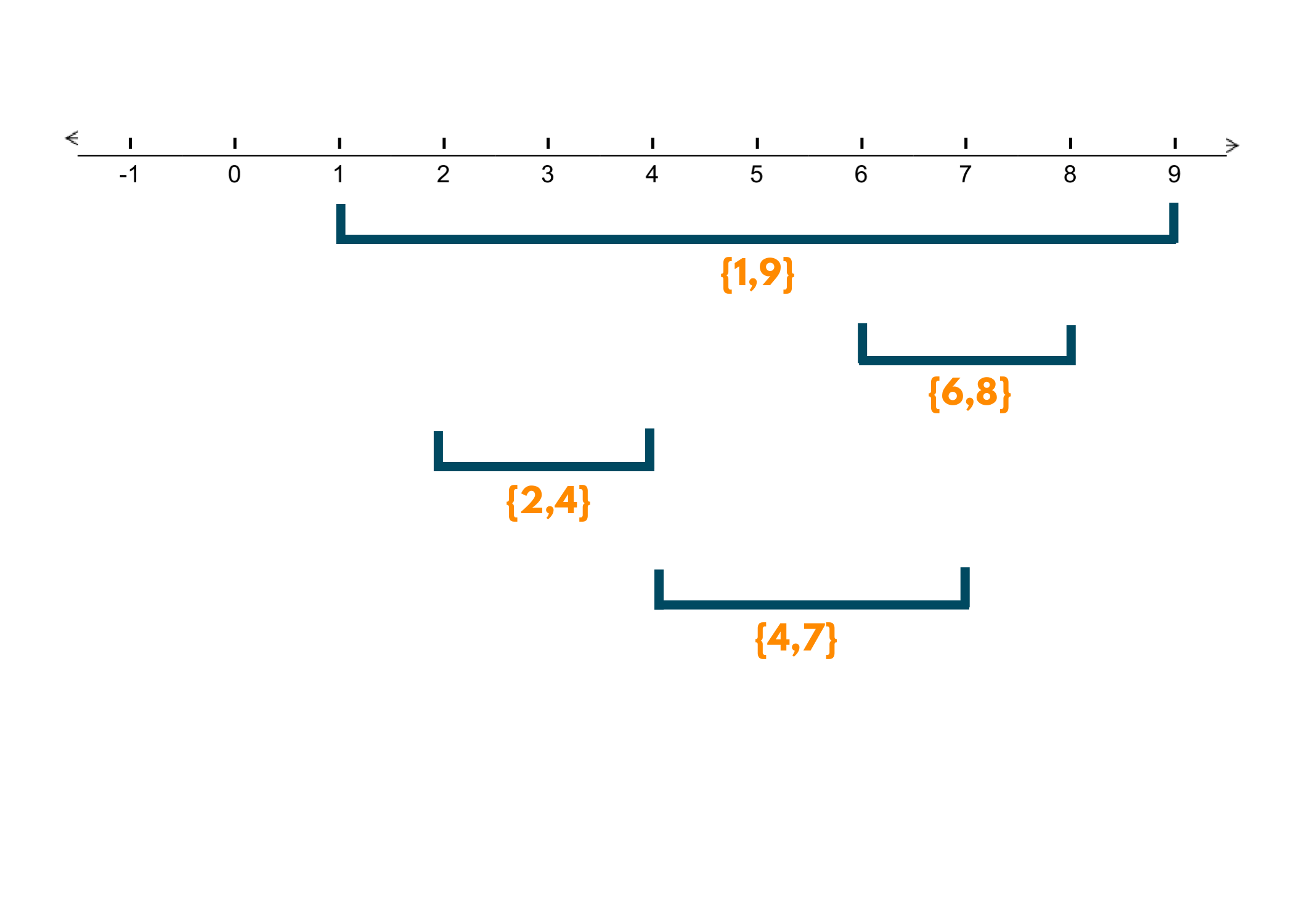Implementation with C++ Code:

```//C++ Program for Merge Intervals
#include<bits/stdc++.h>
using namespace std;

vector<vector<int>> MergeIntervals(vector<vector<int>>& intervals) {
//Storing merged intervals in this array
vector<vector<int>> ans;
//Sorting intervals array according to ascending order
sort(intervals.begin(),intervals.end());
//Temperary array for storing current merged interval
vector<int> temp = intervals;

//Looping from index 1 till nth index
for(int i=1;i<intervals.size();i++){
//if ith interval first element is less than the temp array second element
//then merge into temp array
if(intervals[i]<=temp){
temp = max(temp,intervals[i]);  //Max of both second element
}
//else add the temp array into ans and update temp array into ith interval
else{
ans.push_back(temp);
temp = intervals[i];
}
}
//store the left temp merge array into ans
ans.push_back(temp);

return ans;
}

int main() {
//Initializing the given intervals
vector<vector<int>> intervals = {{1,3},{2,4},{6,8},{7,11},{9,10},{12,15}};

//Function Call For merge Intervals
vector<vector<int>> ans = MergeIntervals(intervals);

//Printing the merged interval array
cout<<"Merged Intervals Are :"<<endl;
for(int i=0;i<ans.size();i++){
cout<<"["<<ans[i]<<","<<ans[i]<<"] ";
}
}
```

Output:

```Merged Intervals Are :
[1,4] [6,11] [12,15]
```

### 10) Two City Scheduling

Problem Statement: 2n people are getting interviewed by a company. You are given array costs[] that contains [aCost[i], bCosr[i]]. The cost of flying a person to city is aCost[i] and that of flying him to city is bCost[i]. You need to find the minimum cost to fly people to a city such that people arrive in each city.

Approach toward Problem: Since there are 2n people and you need people in every city, this means that half people will go to the city and another half to city b. Now, as you are given the flying costs of people for both the cities. So, you need to find the minimum cost of going to a particular city for a person. How to do that?

1. Sort the given costs in ascending order.
2. Now, you need half people in one city and another half in the second.
3. So, divide the total number of people by 2. The initial half will go to city and later will visit city b.

Example:

Input: [[10,20],[30,200],[400,50],[30,20]]

Output: 110

Explanation: When we sort the array in ascending order, we get [[10,20],[30,200],[30,20], [400,50]]. So, the first two will visit the city hence, the result will be 10+30=40 and the latter will go to city therefore, costing 50+20=70. Therefore, 40+70= 110

Implementation with C++ Code:

```//C++ Program for Two city Scheduling
#include<bits/stdc++.h>
using namespace std;

//Comparison of array to sort according to greater diff comes first
bool static relativeCost(vector<int>& first,vector<int>& second){
return first-first > second-second;
}

int twoCitySchedCost(vector<vector<int>>& costs) {
int sum = 0;   // to store the minCost

//Sorting the cost array according to min cost of city a on first half and min cost of city b on second       half
sort(costs.begin(),costs.end(), relativeCost);

//costs of interviewing in city a
for(int i=0;i<costs.size()/2;i++){
sum += costs[i];
}
//Costs for interviewing in city b
for(int i=costs.size()/2;i<costs.size();i++){
sum += costs[i];
}

return sum;
}

int main() {
//Cost for the ith person to interview in city a and b
vector<vector<int>> costs = {{259,770},{448,54},{926,667},{184,139},{840,118},{577,469}};

//Function Call
int minCost = twoCitySchedCost(costs);

//Printing minimum cost to interview half the people in each city
cout<< "The total minimum cost is "<<minCost;
}
```

Output:

```The total minimum cost is 1859
```

## Conclusion

Major tech companies are focusing on optimization due to a rapid decrease in resources and time. A greedy Algorithm, undoubtedly, helps in such scenarios to get the best locally optimal solution with less time complexity. Although, in some cases, a globally optimized solution is needed, still, there is a good scope for solving problems like the above using this approach. It works well for a wide range of problems. Therefore, practicing such questions can prove to be a boon for your placements!

### FavTutor - 24x7 Live Coding Help from Expert Tutors!